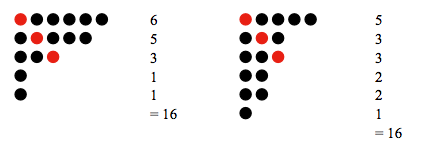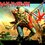# Introduction to partitioning of integers(Part 1)As this is my first note for the Torque Group any feed back from the staff and also my fellow users is welcome.

$\textbf{Q.}$Let $n$ be a positive integer.In how many ways can one write a sum of (at least two) positive integers that add up to n?Consider the same set of integers written in different order to be different.

$\textbf{Ans.}$ Consider the $n-1$ spaces between $n$ 1's in the following arrangement : 1_1_1_1........_1

In each of the spaces either we put "+" or we put ")+(".The number of ways to do it is $2^{n-1}$.It is to be noted that each of these arrangements correspond to a unique way of partitioning n.This happens because by order of operations we need to add the numbers between each pair of parentheses first.

For example, for $n=8$, $(1+1)+(1+1)+(1)+(1+1+1) = 2+2+1+3 = 8$

Among the different possible combination the only one that does not correspond to a legal sum is = $(1+1+1+1+1+...+1)=n$.

So the total number of possible ways is $2^{n-1}-1$

A much more interesting idea is the number of ways in which one can write a sum of integers that add up to n, if different orders of summands are not distinguished.In this series of post I will try to provide a overview of this topic while introducing a few techniques for dealing with them.This will hopefully shed some light on the beauty of the subject for you.

$\textbf{Definition:-}$A partition of an integer n , is one way of writing n as a sum of positive integers where order of the addends(terms to be added) does not matter.For the integer $n$ the function giving the number of partitions is denoted by $p(n)$.

As shown below there are 7 partitions of 5.Thus $p(5) = 7$.

$5 = 5$ $5 = 4+1$ $5 = 3+2$ $5 = 3+1+1$ $5 = 2+2+1$ $5 = 2+1+1+1$ $5 = 1+1+1+1+1$

$\textbf{Ferrer's Diagram(A pictorial representation):-}$A Ferrer's diagram is a way of visualizing partitions with dots.Each row represents one addend in a partition.For example ,The partition of $16$ into $6+5+3+1+1$ and into $5+3+3+2+2+1$ is shown in the given diagram.

Here are a few problems that can be solved using Ferrer's diagrams please post your solutions in the comments.

$\textbf{Problem 1}$. Show that the number of partitions of an integer $n$ into parts the largest of which is $r$ is equal to the number of partitions of $n$ into exactly r parts.

$\textbf{Problem 2}$. Prove that every number of the form $n^{k}$ where $n$ is an integer greater than one and $k$ is a positive integer can be represented as the sum of $n$ positive odd integers.

$\textbf{Problem 3}$. Prove that the number of partitions of $n$ with no part greater than $k$ is equal to the number of partitions of $n + k$ with exactly $k$ parts

In the next post I will introduce generating functions in association to solving partition problems and in another post I will discuss stacking problems.Good Day!!

Click here for part 2Note by Eddie The Head
7 years, 4 months ago

This discussion board is a place to discuss our Daily Challenges and the math and science related to those challenges. Explanations are more than just a solution — they should explain the steps and thinking strategies that you used to obtain the solution. Comments should further the discussion of math and science.

When posting on Brilliant:

• Use the emojis to react to an explanation, whether you're congratulating a job well done , or just really confused .
• Ask specific questions about the challenge or the steps in somebody's explanation. Well-posed questions can add a lot to the discussion, but posting "I don't understand!" doesn't help anyone.
• Try to contribute something new to the discussion, whether it is an extension, generalization or other idea related to the challenge.
• Stay on topic — we're all here to learn more about math and science, not to hear about your favorite get-rich-quick scheme or current world events.

MarkdownAppears as
*italics* or _italics_ italics
**bold** or __bold__ bold
- bulleted- list
• bulleted
• list
1. numbered2. list
1. numbered
2. list
Note: you must add a full line of space before and after lists for them to show up correctly
paragraph 1paragraph 2

paragraph 1

paragraph 2

[example link](https://brilliant.org)example link
> This is a quote
This is a quote
    # I indented these lines
# 4 spaces, and now they show
# up as a code block.

print "hello world"
# I indented these lines
# 4 spaces, and now they show
# up as a code block.

print "hello world"
MathAppears as
Remember to wrap math in $$ ... $$ or $ ... $ to ensure proper formatting.
2 \times 3 $2 \times 3$
2^{34} $2^{34}$
a_{i-1} $a_{i-1}$
\frac{2}{3} $\frac{2}{3}$
\sqrt{2} $\sqrt{2}$
\sum_{i=1}^3 $\sum_{i=1}^3$
\sin \theta $\sin \theta$
\boxed{123} $\boxed{123}$

## Comments

Sort by:

Top Newest

Can anyone tell me how to place a picture in a definite spot in the post and also attaching multiple images...Thanks in advance....

- 7 years, 4 months ago

Log in to reply

I don't even know how to attach a picture. My computer doesn't let me click on the link to attach a picture. :/

- 7 years, 4 months ago

Log in to reply

@Finn Hulse do

![random text here](image link here)


Notice the exclaimation mark. REMEMBER NO SPACES.

- 7 years, 4 months ago

Log in to reply

So... How do I figure out how many ways I can add numbers to get a number? You never said a formula.

- 7 years, 4 months ago

Log in to reply

In this post I have defined the pictorial representation only ..the main formula will be using generating functions in my next post,,,the pictorial representation itself cab be used to prove a few properties...Here is part 2

- 7 years, 4 months ago

Log in to reply

What do u mean in Problem 1?

- 7 years, 4 months ago

Log in to reply

Prove that the number of ways to partition a number $n$ such that the largest partition of it has value $r$ is the same as the number of partitions of $n$ into $r$ partitions. Does that make any more sense?

- 7 years, 4 months ago

Log in to reply

yes! Thanks Daniel L. :)

- 7 years, 4 months ago

Log in to reply

I think the problem is judt as it says....try on a smaller example to get a better idea

- 7 years, 4 months ago

Log in to reply

Can anyone explain why $(1+1+...+1) = n$ is not a legal sum ?

- 7 years, 3 months ago

Log in to reply

In the question we are required to find the no of sums of at least 2 two positive integers that add up to n... (1+1+1+1....+1) corresponds to the sum n+0 and 0 is not positive...

- 7 years, 3 months ago

Log in to reply

I am not even sure what I am writing is even sane, but I think the answer to problem lies in the fact that nC(n-r) is equal to nCr(where aCb = a!/((a-b)!.b!)). Also (1+1)^n=1+n....n+1. As the largest number possible is r therefore there are exactly (n/r)-1 minimum number of spaces between the additions(where (n/r) is the closest larger integer if n/r isn't an integer and denotes simple bracket if it an integer). Therefore, by simple combinatorics and using my first statement I think both are equal(2 to power((n/r)-1)-1. I would request you to make sense of my answer if you can,for I am not as good as you are in explaining things to others and must confess that I have an intuitive idea of Problem 1 at best. If I am wrong altogether, then please write the correct solution so that I can understand how it works

- 7 years, 3 months ago

Log in to reply

×

Problem Loading...

Note Loading...

Set Loading...